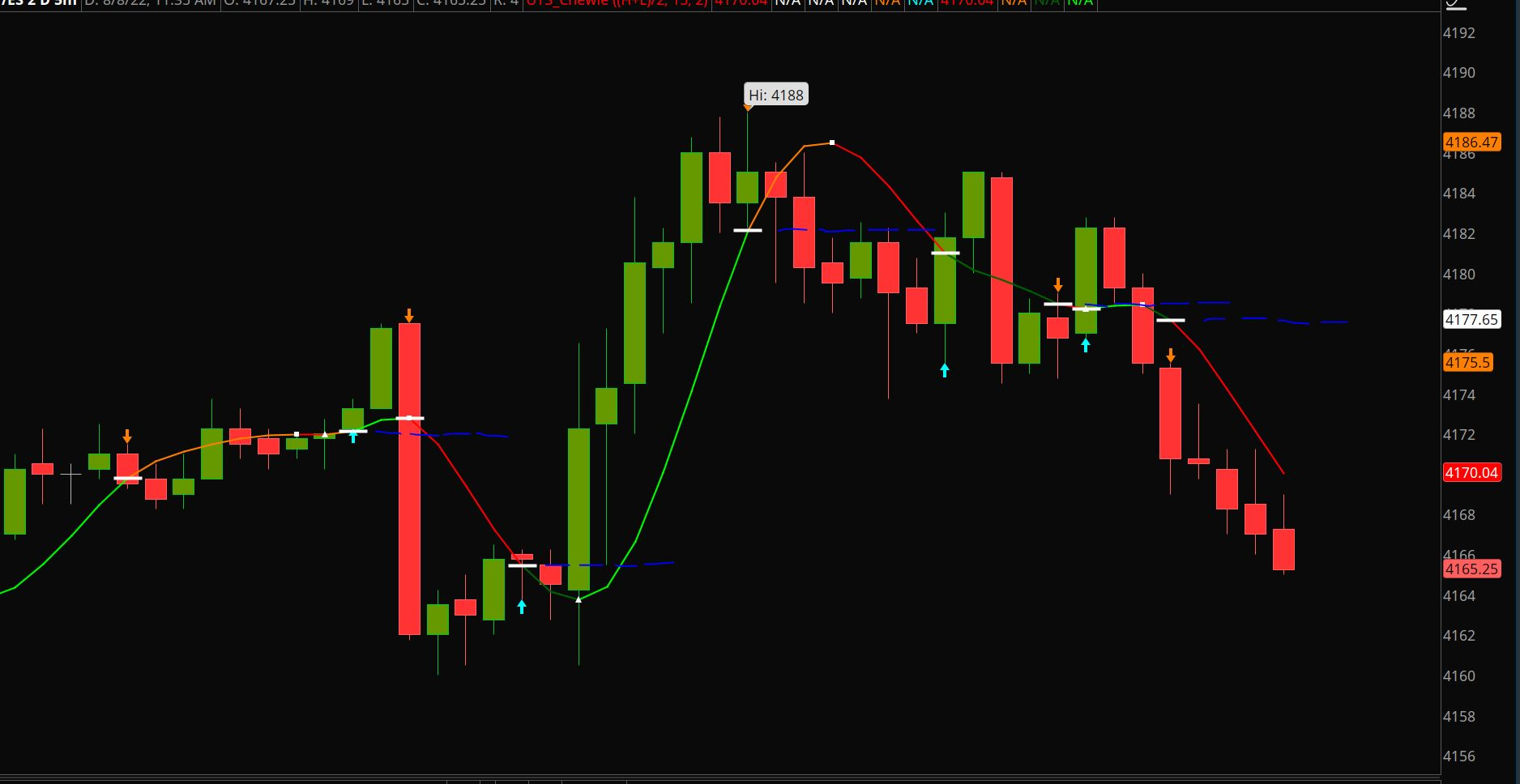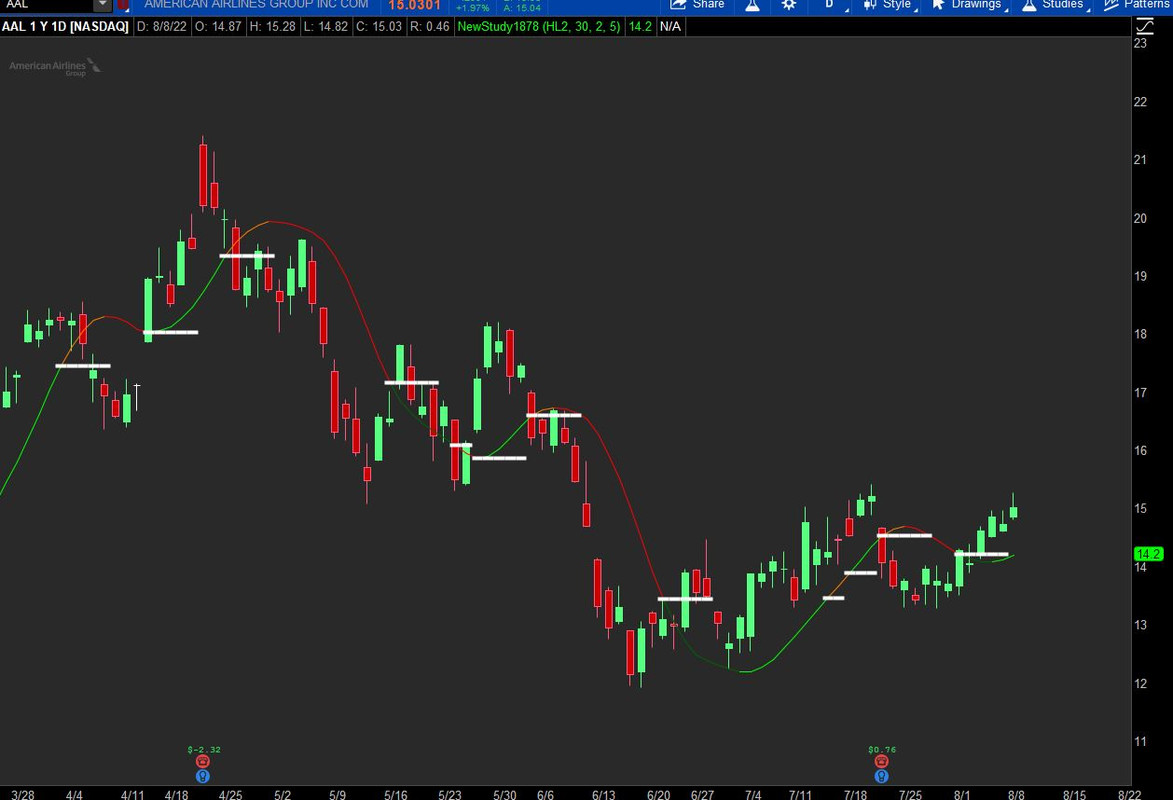#### 8Nick8

##### Active member
2019 Donor
VIP
declare upper;
input price = HL2;
input HMA_Length = 30;
input lookback = 2;
plot HMA = HullMovingAvg(price = price, length = HMA_Length);
def delta = HMA - HMA[lookback + 1];
def delta_per_bar = delta / lookback;
def next_bar = HMA + delta_per_bar;
def concavity = if HMA > next_bar then 1 else -1;
plot turning_point = if concavity != concavity then HMA else double.nan;

HMA.AssignValueColor(color = if concavity == -1 then
if HMA > HMA then color.dark_orange else color.red else
if HMA < HMA then color.dark_green else color.green);

HMA.SetLineWeight(1);
turning_point.SetLineWeight(4);
turning_point.SetPaintingStrategy(paintingStrategy.Horizontal);
turning_point.SetDefaultColor(color.white);

https%3A//i.imgur.com/4WqZonz.jpg[/img]']Hi, I wanted to plot 5 dashes whenever the turning pt conditions are met. I have changed the plot to "horizontal" but can only get 1 dash. Can some one help to correct my error. Thanks

declare upper;
input price = HL2;
input HMA_Length = 30;
input lookback = 2;
plot HMA = HullMovingAvg(price = price, length = HMA_Length);
def delta = HMA - HMA[lookback + 1];
def delta_per_bar = delta / lookback;
def next_bar = HMA + delta_per_bar;
def concavity = if HMA > next_bar then 1 else -1;
plot turning_point = if concavity != concavity then HMA else double.nan;

HMA.AssignValueColor(color = if concavity == -1 then
if HMA > HMA then color.dark_orange else color.red else
if HMA < HMA then color.dark_green else color.green);

HMA.SetLineWeight(1);
turning_point.SetLineWeight(4);
turning_point.SetPaintingStrategy(paintingStrategy.Horizontal);
turning_point.SetDefaultColor(color.white);

15389[/ATTACH]']Hi, I wanted to plot 5 dashes whenever the turning pt conditions are met. I have changed the plot to "horizontal" but can only get 1 dash. Can some one help to correct my error. Thanks

First count is defined to start when concavity!=concavity. The turningpoint is first defined as an extended line in the def tp. Then turningpoint is limited to the length input, defaulted to 5.Ruby:
``````declare upper;
input price = HL2;
input HMA_Length = 30;
input lookback = 2;
plot HMA = HullMovingAvg(price = price, length = HMA_Length);
def delta = HMA - HMA[lookback + 1];
def delta_per_bar = delta / lookback;
def next_bar  = HMA + delta_per_bar;
def concavity = if HMA > next_bar then 1 else -1;

def count          = if concavity != concavity then 1 else count + 1;
def tp             = if IsNaN(close)
then tp
else if concavity != concavity
then HMA
else tp;
input length       =  5;
plot turning_point = if Between(count, 1, length) then tp else Double.NaN ;

HMA.AssignValueColor(color = if concavity == -1 then
if HMA > HMA then Color.DARK_ORANGE else Color.RED else
if HMA < HMA then Color.DARK_GREEN else Color.GREEN);

HMA.SetLineWeight(1);
turning_point.SetLineWeight(4);
turning_point.SetPaintingStrategy(PaintingStrategy.HORIZONTAL);
turning_point.SetDefaultColor(Color.WHITE);``````

#### Attachments

•8Nick8

### Not the exact question you're looking for?

87k+ Posts
302 Online## The Market Trading Game Changer

Join 2,500+ subscribers inside the useThinkScript VIP Membership Club
• Exclusive indicators
• Proven strategies & setups
• Private Discord community
• Exclusive members-only content
• 1 full year of unlimited support

What is useThinkScript?

useThinkScript is the #1 community of stock market investors using indicators and other tools to power their trading strategies. Traders of all skill levels use our forums to learn about scripting and indicators, help each other, and discover new ways to gain an edge in the markets.

How do I get started?

We get it. Our forum can be intimidating, if not overwhelming. With thousands of topics, tens of thousands of posts, our community has created an incredibly deep knowledge base for stock traders. No one can ever exhaust every resource provided on our site.

If you are new, or just looking for guidance, here are some helpful links to get you started.

What are the benefits of VIP Membership?